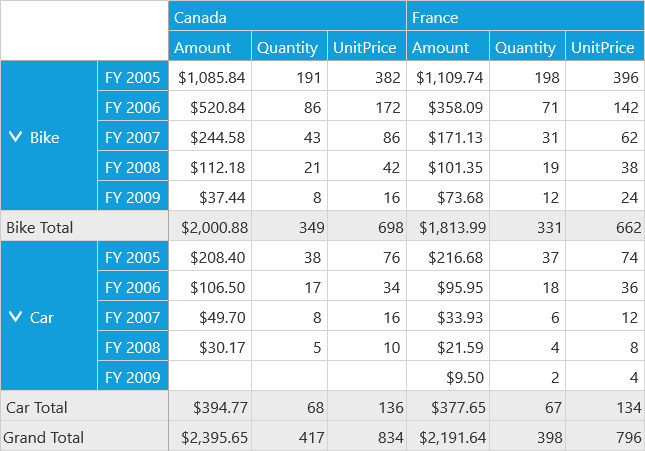# Calculated Field in UWP Pivot Client (SfPivotClient)

10 May 20211 minute to read

The SfPivotClient supports adding a new calculated field based on existing calculated items using the calculated field dialog.

1. To add a calculated field, click the Calculated Field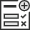in the client toolbar. The calculated field dialog opens.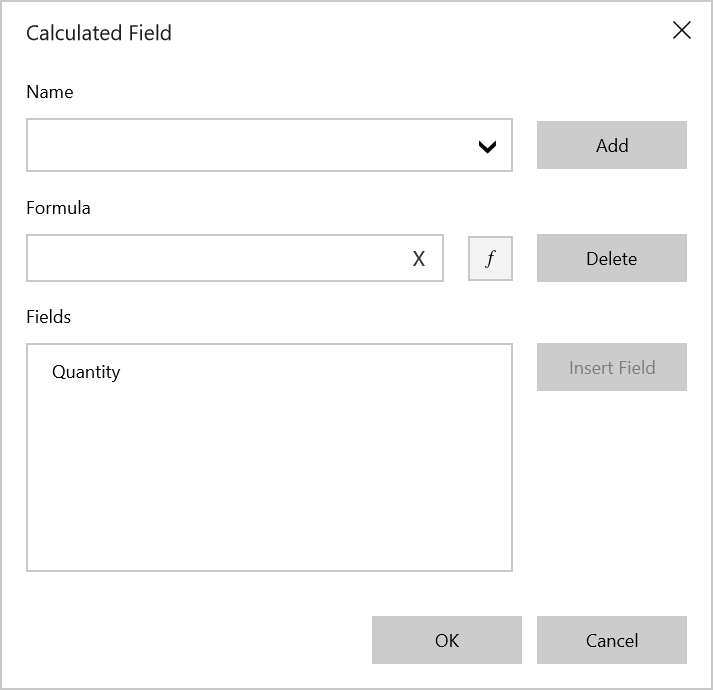1. Define the Name for the calculated field.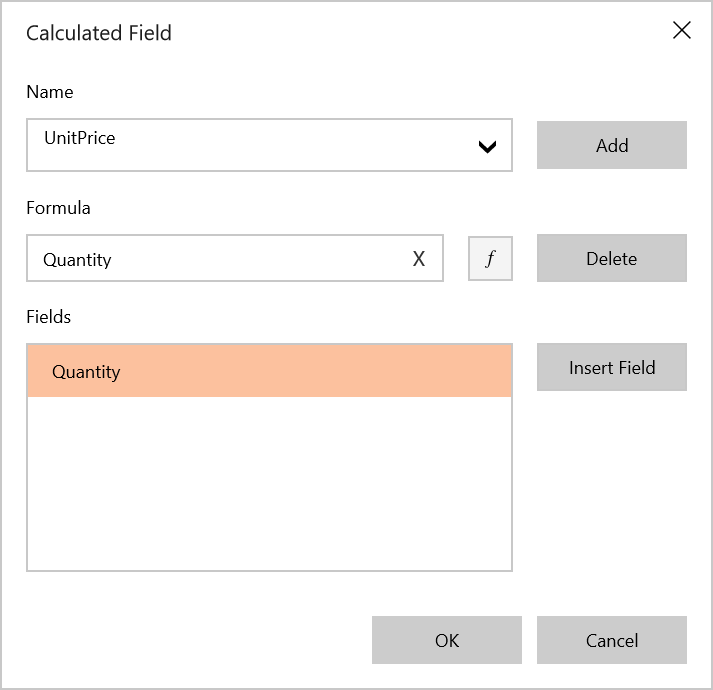1. Enter the Formula by inserting the calculation fields through the Fields section. For inserting numerical operators, you can use the formula pop-up as shown in the following screenshot.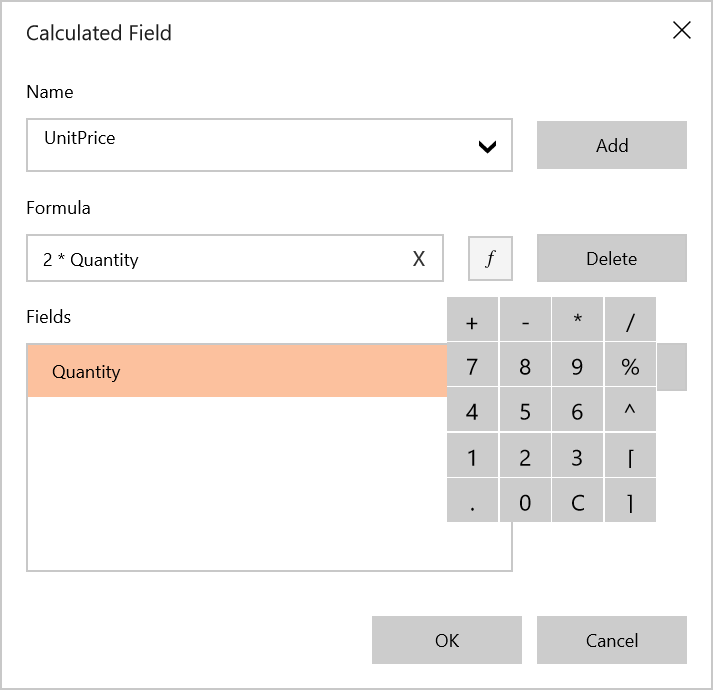1. Click Add to add the calculated field, and then click OK to populate the values in the SfPivotClient.